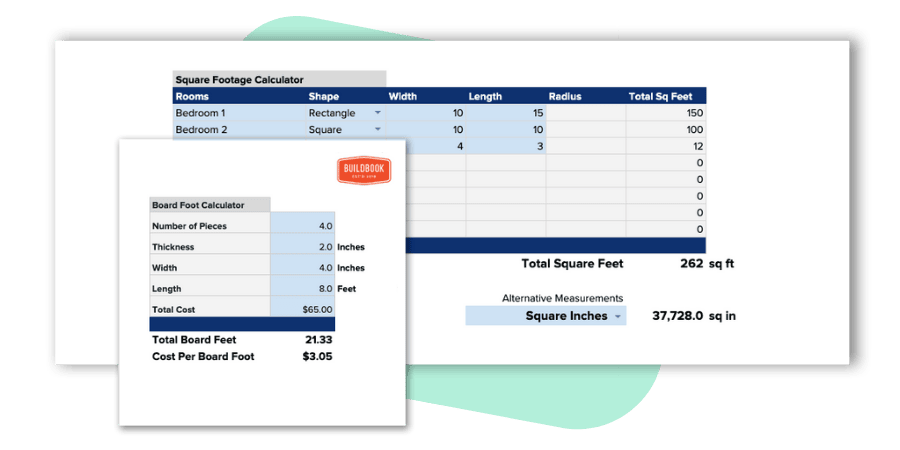# Square Footage CalculatorFind the total square footage of a square, rectangular, circular, or triangular area

## Want an even better square footage calculator with more measuring tools?

This collection of construction calculators is 100% free to download, copy, and use as your own. You're free to modify and/or adjust any calculator to best fit the needs of you or your business.This square footage calculator allows you to find the total square footage of a square, rectangular, circular, or triangular area using basic dimensions in feet.

## How to calculate square footage of an area

By all accounts, square footage is the most common measurement used to define the size of an area within residential construction projects in North America and beyond. It will often be described as, the house has 2,500 finished square feet, or the master bedroom is 200 square feet. In a square or rectangle area, the square footage is determined by simply multiplying the length by the width in feet. A single unit in square footage is 1 square foot, which is a square area measuring 1 foot in length by 1 foot in width.

The shape of the area in which you're measuring will determine which of the following formulas you should use to calculate the total square feet.

#### Square or Rectangle Area

Length (ft) x Width (ft) = Total Square Feet

#### Circular Area

3.14 x (Diameter (ft)/2)^2 = Total Square Feet

#### Triangular Area

1/2 x Length of Side 1 (ft) * Length of Side 2 (ft) = Total Square Feet

## How to measure the square footage of a house

Most often you'll hear the size of a home be described in finished square feet. This simply means that this is the total area of the completed portions of a home. For example, if a home includes a basement that hasn't been completed, the area of that basement would not be included as part of the finished square feet.

To find the square footage of a home, first determine if you'd like to find the finished square feet or the total square feet.

Once you've decided which measurement you're after, you'll want to break down your home by rooms/areas to make the calculations of each area easier to manage. i.e. Bedroom 1, Bedroom 2, Living Room, etc.

For each room, you'll need to measure the width and length in feet, including any closet space, nooks, entry ways, etc. The simplest way to accomplish this is to break down each room/area into smaller areas in regular shapes. For example, measure the closet separately from the main area of a bedroom. When each of these smaller areas within a room are measured, you'll calculate the square footage of each and add them all together to get the total square footage of the bedroom.